# Sentence cluster with Kmeans algorithm

This is unsupervised learning project in the SJSU data mining course. Students need to classify the sentences (already numerized by the SJSU course Prof) into clusters using Kmeans algorithm. Below is the performance of students in the class. Seems not so bad.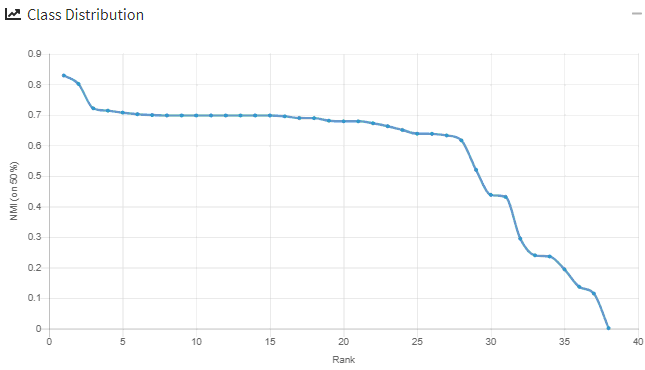The whole project includes 3 parts: data preprocess, Bisec Kmeans and the basic Kmeans algorithm.

1. Data preprocess

The clustering documents have 27673 terms in total. That is said number of features are 27673. Here is an excerpt of the sample data.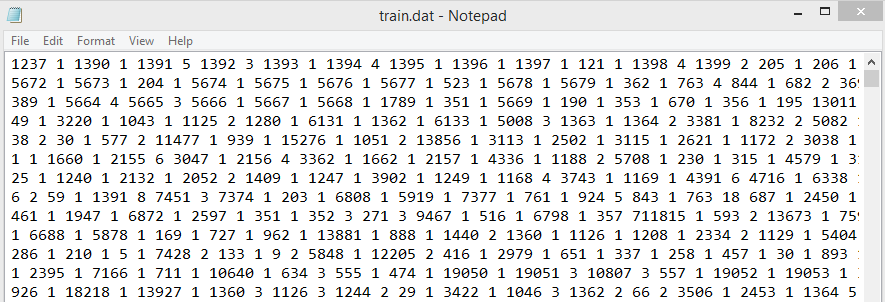First, we will build a sparse matrix to re-index all terms in the documents. Then, we need to identify the importance of each term in each document.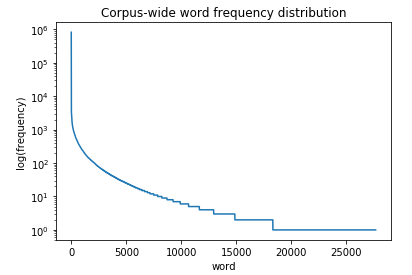It is easy to find out that term “1” is occurred in all those documents. That means “1” is useless for our cluster analysis. Here, I will use the TF-IDF to identify the importance of words in the documents.  The TF-IDF value is used in our text mining as a weight for each term. This weight is a good measure to evaluate how important a term in the document.As the curse of high dimension, we need to reduce the dimension via the LSA (i.e truncated SVD). The reason is that “”Nearly all of the high-dimensional space is “far away” from the centre. To put it another way, the high-dimensional unit hypercube can be said to consist almost entirely of the “corners” of the hypercube, with almost no “middle”.”””

We use the truncated SVD to shrink the origianl number of features from 27673 to 5000, so that it still capture the 95% of variance after the procedure.As our Kmeans algorithm will use the cosine similarity as the measurement for cluster. We need to normalize each record with L2 norm equal to 1. This will help the efficiency of cosine similarity calculation. Below is the formula for two documents A, B.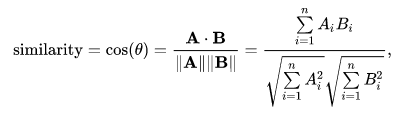The training data is generated based on the frequency of each word/term shown in each sentence. Here is the code.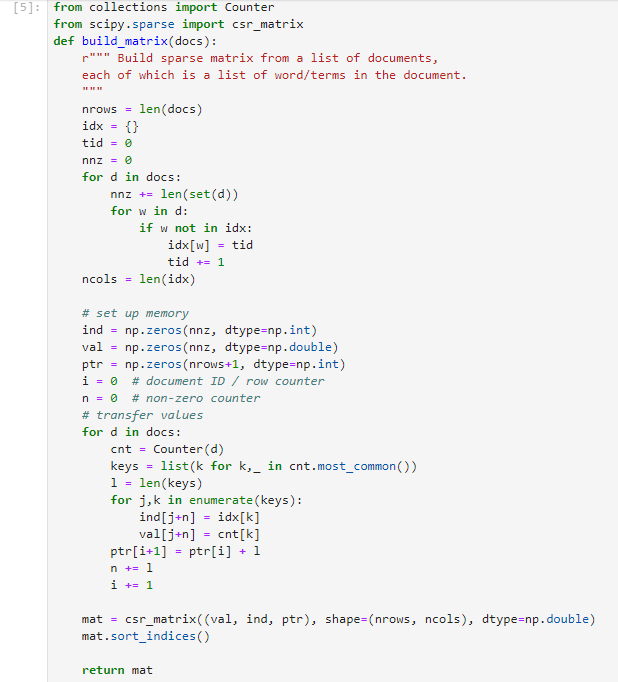2. Bisec Kmeans algorithm

We use the SSE to measure how well after adding one cluster into the data.  SSE is the sum of distance of each point to the each centroid.  Here is the Bisec Kmeans algorithm.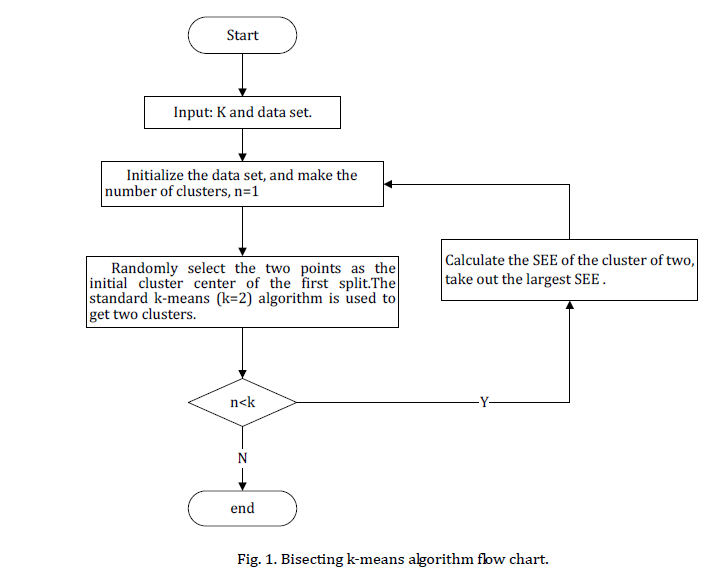3. Basic Kmeans algorithm (with number of Clusters equal to 2 used by above Bisec Kmeans)

As mentioned above, we will use the cosine similarity as the measurement to form a cluster. For each sentence, we will calculate the distance between two centroids, and assign the sentence into the shortest cluster. After all sentences clustered, it will have 2 new centroids, and repeat the process until no change of centroid. Below is the algorithm summary.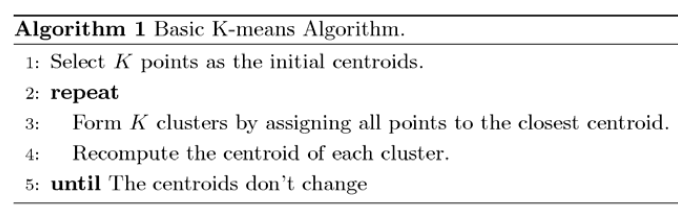4. Extra try.

I try the tsne to further dimension reduction to 8. But the result is similar.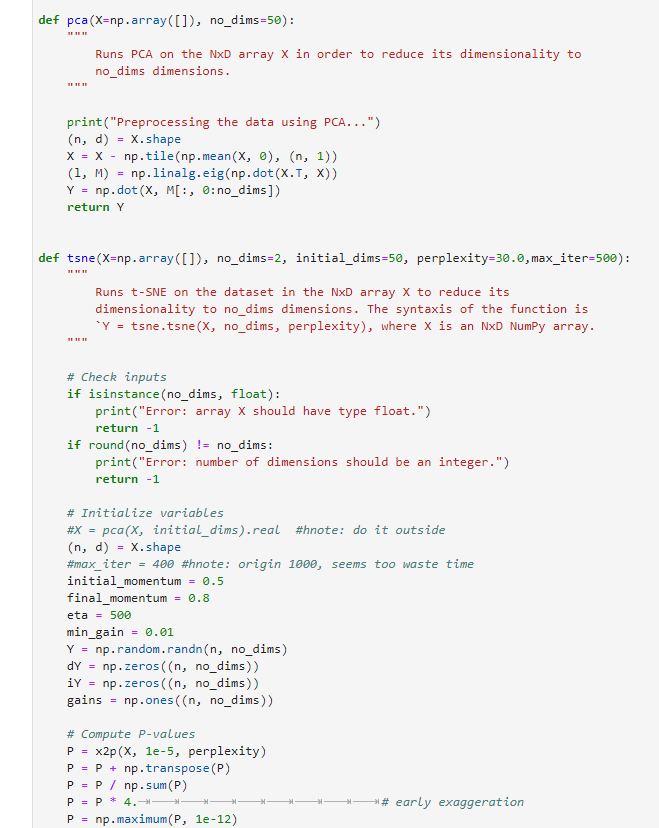5. The metrics for evaluating clustering

We will use the SSE to evaluate each round of cluster, also to compare different methods (such as with/without LSA, with/without tsne)

Here is the kmeans gain result from cluster number 1 to 7.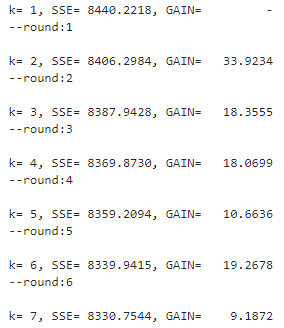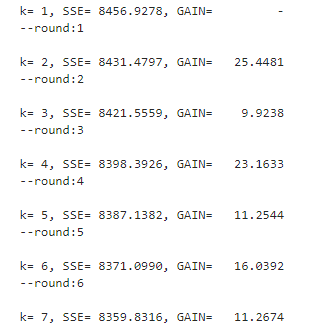When handling LSA, seems we do not need to have too big cumulated variance. In the first run, I try cumulated variance with 0.87, which look like better than cumulated variance with 0.95.

6. The result

NMI is top 25%. The project result seems ok. In this project, I implement the iterative Bisec Kmeans algorithm with basic Kmeans as base.

« (Previous News)